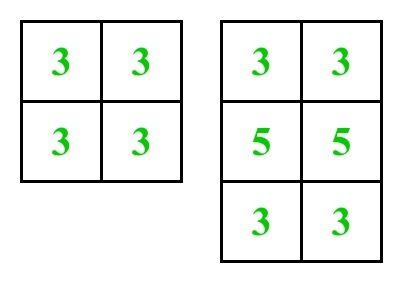# Sum of the count of number of adjacent squares in an M X N grid

Given an M × N matrix. The task is to count the number of adjacent cells and calculate their sum.

Two cells are said to be connected if they are adjacent to each other horizontally, vertically, or diagonally.

Examples :

Input : m = 2, n = 2
Output : 12

Input : m = 3, n = 2
Output: 22

See the below diagram where numbers written on it denotes number of adjacent squares.## Recommended: Please try your approach on {IDE} first, before moving on to the solution.

Approach:

In a m X n grid there can be 3 cases:

• Corner cells touch 3 cells, and there are always 4 corner cells.
• Edge cells touch 5 cells, and there are always 2 * (m+n-4) edge cells.
• Interior cells touch 8 cells, and there are always (m-2) * (n-2) interior cells.

Therefore,

```Sum = 3*4 + 5*2*(m+n-4) + 8*(m-2)*(n-2)
= 8mn - 6m - 6n +4
```

Below is the implementation of the above approach:

## C++

 `// C++ implementation of the above approach ` ` `  `#include ` `using` `namespace` `std; ` ` `  `// function to calculate the sum of all cells adjacent value ` `int` `sum(``int` `m, ``int` `n) ` `{ ` `    ``return` `8 * m * n - 6 * m - 6 * n + 4; ` `} ` ` `  `// Driver program to test above ` `int` `main() ` `{ ` `    ``int` `m = 3, n = 2; ` `    ``cout << sum(m, n); ` ` `  `    ``return` `0; ` `} `

## Java

 `// Java implementation of the above approach  ` `class` `GFG  ` `{ ` `     `  `    ``// function to calculate the sum  ` `    ``// of all cells adjacent value  ` `    ``static` `int` `sum(``int` `m, ``int` `n)  ` `    ``{  ` `        ``return` `8` `* m * n - ``6` `* m - ``6` `* n + ``4``;  ` `    ``}  ` `     `  `    ``// Driver Code ` `    ``public` `static` `void` `main (String[] args) ` `    ``{  ` `        ``int` `m = ``3``, n = ``2``;  ` `        ``System.out.println(sum(m, n));  ` `    ``}  ` `} ` ` `  `// This Code is contributed by AnkitRai01 `

## Python3

 `# Python3 implementation of the above approach ` ` `  `# function to calculate the sum ` `# of all cells adjacent value ` `def` `summ(m, n): ` `    ``return` `8` `*` `m ``*` `n ``-` `6` `*` `m ``-` `6` `*` `n ``+` `4` ` `  `# Driver Code ` `m ``=` `3` `n ``=` `2` `print``(summ(m, n)) ` ` `  `# This code is contributed by Mohit Kumar `

## C#

 `// C# implementation of the above approach  ` `using` `System; ` `class` `GFG  ` `{ ` `     `  `    ``// function to calculate the sum  ` `    ``// of all cells adjacent value  ` `    ``static` `int` `sum(``int` `m, ``int` `n)  ` `    ``{  ` `        ``return` `8 * m * n - 6 * m - 6 * n + 4;  ` `    ``}  ` `     `  `    ``// Driver Code ` `    ``public` `static` `void` `Main (String []args) ` `    ``{  ` `        ``int` `m = 3, n = 2;  ` `        ``Console.WriteLine(sum(m, n));  ` `    ``}  ` `} ` ` `  `// This code is contributed by andrew1234 `

Output:

```22
```

Time Complexity:Attention reader! Don’t stop learning now. Get hold of all the important DSA concepts with the DSA Self Paced Course at a student-friendly price and become industry ready.

My Personal Notes arrow_drop_upCheck out this Author's contributed articles.

If you like GeeksforGeeks and would like to contribute, you can also write an article using contribute.geeksforgeeks.org or mail your article to contribute@geeksforgeeks.org. See your article appearing on the GeeksforGeeks main page and help other Geeks.

Please Improve this article if you find anything incorrect by clicking on the "Improve Article" button below.

Article Tags :
Practice Tags :

1

Please write to us at contribute@geeksforgeeks.org to report any issue with the above content.